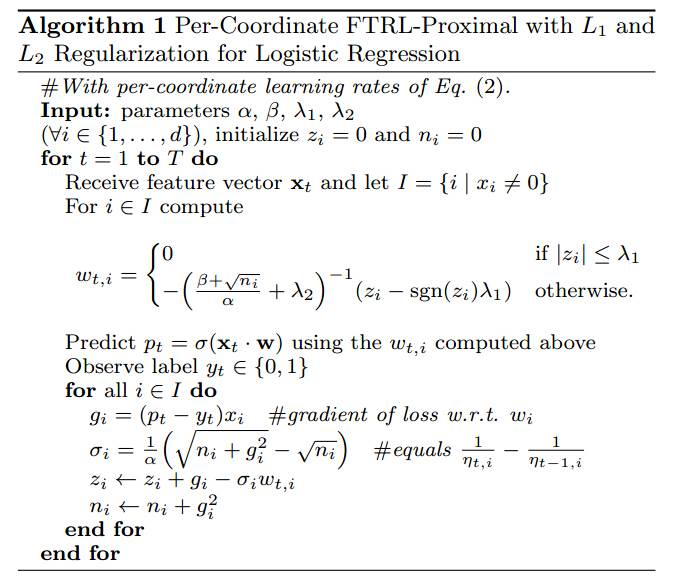# FM, FTRL, Softmax

## 前言

最近公司内部举办了一届数据挖掘大赛，题目是根据用户的一些属性和行为数据来预测性别和年龄区间，属于一个二分类问题（性别预测男女）和一个多分类问题（年龄分为7个区间），评判标准为logloss。共有五六十支队伍提交，我们组的三名小伙伴最终取得第三名的好成绩，跟前两名只有千分之一二的差距。

赛后总结，发现前6名全部使用了DNN模型，而我们团队比较特别的是，不只使用了DNN，还有FM，最终方案是六七个DNN模型和一个FM模型的ensembling。

其实比赛刚开始，他们使用的是XGBoost，因为XGBoost的名头实在太响。但这次比赛的数据量规模较大，训练样本数达到千万，XGBoost跑起来异常的慢，一个模型要跑一两天。于是我把几个月前写的FM工具给他们用，效果非常好，二分类只需十几分钟，多分类也就半个多小时，logloss和XGBoost基本持平，甚至更低。最终他们抛弃了XGBoost，使用FM在快速验证特征和模型融合方面都起到了很好的作用。此外，我们组另外两名实习生仅使用此FM工具就取得了第七名的成绩。

最初写此FM代码时正值alphaGo完虐人类，因此随手给这个工具起了个名字叫alphaFM，今天我就来分享一下这个工具是如何实现的。

## alphaFM介绍

实现alphaFM的初衷是解决大规模数据的FM训练，在我们真实的业务数据中，训练样本数常常是千万到亿级别，特征维度是百万到千万级别甚至上亿，这样规模的数据完全加载到内存训练已经不太现实，甚至下载到本地硬盘都很困难，一般都是经过spark生成样本直接存储在hdfs上。

alphaFM用于解决这样的问题特别适合，一边从hdfs下载，一边计算，一个典型的使用方法是这样：

训练：10个线程计算，factorization的维度是8，最后得到模型文件fm_model.txt

hadoop fs -cat train_data_hdfs_path | ./fm_train -core 10 -dim 1,1,8 -m fm_model.txt

测试：10个线程计算，factorization的维度是8，加载模型文件fm_model.txt，最后输出预测结果文件fm_pre.txt

hadoop fs -cat test_data_hdfs_path | ./fm_predict -core 10 -dim 8 -m fm_model.txt -out fm_pre.txt

当然，如果样本文件不大，也可以先下载到本地，然后再运行alphaFM。

由于采用了FTRL，调好参数后，训练样本只需过一遍即可收敛，无需多次迭代，因此alphaFM读取训练样本采用了管道的方式，这样的好处除了节省内存，还可以通过管道对输入数据做各种中间过程的转换，比如采样、格式变换等，无需重新生成训练样本，方便灵活做实验。

alphaFM还支持加载上次的模型，继续在新数据上训练，理论上可以一直这样增量式进行下去。

FTRL的好处之一是可以得到稀疏解，在LR上非常有效，但对于FM，模型参数v是个向量，对于每一个特征，必须w为0且v的每一维都为0才算稀疏解， 但这通常很难满足，所以加了一个force_v_sparse的参数，在训练过程中，每当w变成0时，就强制将对应的v变成0向量。这样就可以得到很好的稀疏效果，且在我的实验中发现最终对test样本的logloss没有什么影响。

当将dim参数设置为1,1,0时，alphaFM就退化成标准的LR的FTRL训练工具。不禁想起我们最早的LR的FTRL代码还是勇保同学写的，我现在的代码基本上还是沿用了当初的多线程思路，感慨一下。

alphaFM能够处理的特征维度取决于内存大小，训练样本基本不占内存，理论上可以处理任意多的数量。后续可以考虑基于ps框架把alphaFM改造成分布式版本，这样就可以支持更大的特征维度。

接下来请各位打起精神，我们来推一推公式。诗云，万丈高楼平地起，牛不牛逼靠地基。公式就是算法工具的地基，公式整明白了，像我们这种”精通”C++的（谁简历里不是呢:-P），实现就是分分钟的事（装B中，勿扰：-）。

## 二分类问题

对于二分类，最常见的模型是LR，搭配FTRL优化算法。LR的输出会用到sigmoid函数，定义为：
$$\sigma(x)=\frac{1}{1+e^{-x}}$$
LR预测输入$x$是正样本的概率：
$$P(y=1|x,w)=\frac{1}{1+e^{-w^Tx}}=\sigma(w^Tx)$$
可以看到，$\sigma$函数的参数部分 $w^Tx$ 是一个线性函数，这也就是LR被称作线性模型的原因，模型参数只有一个$w$向量，相对简单。如果我们把这部分弄复杂呢？比如这样：
$$\hat{y}(x|\Theta):=w_0+\sum_{i=1}^nw_ix_i+\sum_{i=1}^n\sum_{j=i+1}^n\langle v_i,v_j\rangle x_ix_j\\ =w_0+\sum_{i=1}^nw_ix_i+\sum_{i=1}^n\sum_{j=i+1}^nx_ix_j\sum_{f=1}^kv_{i,f}v_{j,f}\\ =w_0+\sum_{i=1}^nw_ix_i+\frac{1}{2}\sum_{f=1}^k\left(\left(\sum_{i=1}^nv_{i,f}x_i\right)^2-\sum_{i=1}^nv_{i,f}^2x_i^2\right)$$
其中，$x\in R^n$，$w_0\in R$，$w\in R^n$，$V\in R^{n\times k}$，这其实就是一个2阶FM，模型参数 $\Theta=\{w_0,w_1,…,w_n,v_{1,1},…,v_{n,k}\}$ 。如果直接将 $\hat{y}(x|\Theta)$ 做输出，采用平方损失函数便可解决回归问题。而对于二分类问题，外面套一个sigmoid函数即可：
$$P(y=1|x,\Theta)=\frac{1}{1+e^{-\hat{y}(x|\Theta)}}$$
对于$y\in \{-1,1\}$，可统一成形式：
$$P(y|x,\Theta)=\frac{1}{1+e^{-y\hat{y}(x|\Theta)}}=\sigma(y\hat{y}(x|\Theta))$$
模型参数估计采用最大似然的方法，对于训练数据$S$，最优化问题为：
$$\mathop{\arg\max}_{\Theta}\prod_{(x,y)\in S}P(y|x,\Theta)=\mathop{\arg\min}_{\Theta}\sum_{(x,y)\in S}-\ln P(y|x,\Theta)$$
即样本 $(x,y)$ 的损失函数为：
$$l(\Theta|x,y)=-\ln P(y|x,\Theta)=-\ln \sigma(y\hat{y}(x|\Theta))$$
此损失函数对 $\hat{y}$ 求偏导会得到一个优雅简单的形式：
$$\frac{\partial l}{\partial\hat{y}}=y(\sigma(y\hat{y})-1)$$
再配合上 $\hat{y}$ 对模型参数的偏导：
$$\frac{\partial\hat{y}}{\partial\theta}= \begin{cases} 1, & if\,\,\theta\,\,is\,\,w_0 \\ x_i, & if\,\,\theta\,\,is\,\,w_i \\ x_i\sum_{j=1}^nv_{j,f}x_j-v_{i,f}x_i^2 & if\,\,\theta\,\,is\,\,v_{i,f} \\ \end{cases}$$
便可得到损失函数 $l$ 对所有模型参数的偏导，即：
$$g_0^w=\frac{\partial l}{\partial w_0}=y(\sigma(y\hat{y})-1)\\ g_i^w=\frac{\partial l}{\partial w_i}=y(\sigma(y\hat{y})-1)x_i\\ g_{i,f}^v=\frac{\partial l}{\partial v_{i,f}}=y(\sigma(y\hat{y})-1)(x_i\sum_{j=1}^nv_{j,f}x_j-v_{i,f}x_i^2)$$但其实FTRL是一个online learning的框架，能解决的问题绝不仅仅是LR，已经成了一个通用的优化算子，比如TensorFlow的optimizer中都包含了FTRL。我们只要把截图中的伪代码修改，$p_t$的计算改为 $\hat{y}(x|\Theta)$，对于每一轮的特征向量$x$的每一维非0特征$x_i$，都要相应的更新模型参数$w_0,w_i,v_{i,1},…,v_{i,k}$，更新公式不变和截图一致，梯度$g$的计算即为损失函数对每个参数的偏导，前面已经给出。$\sigma,z,n$的更新公式不变。伪代码如下：

Algorithm: alphaFM

$Input:paramters\,\alpha^w,\alpha^v,\beta^w,\beta^v,\lambda_1^w,\lambda_1^v,\lambda_2^w,\lambda_2^v,\sigma$
$Init:w_0=0;n_0^w=0;z_0^w=0;$
$Init:\forall i,\forall f,w_i=0;n_i^w=0;z_i^w=0;v_{i,f}\sim N(0,\sigma);n_{i,f}^v=0;z_{i,f}^v=0;$
$for\,t=1\,to\,T,do$
$\qquad Receive\,feature\,vector\,x\,and\,let\,I=\{i|x_i\neq0\}$
$$w_0= \begin{cases} 0 & if\,\,|z_0^w|\le\lambda_1^w \\ -\left(\frac{\beta^w+\sqrt{n_0^w}}{\alpha^w}+\lambda_2^w\right)^{-1}(z_0^w-sgn(z_0^w)\lambda_1^w) & otherwise. \\ \end{cases}$$
$\qquad for\,i\in I,compute$
$$w_i= \begin{cases} 0 & if\,\,|z_i^w|\le\lambda_1^w \\ -\left(\frac{\beta^w+\sqrt{n_i^w}}{\alpha^w}+\lambda_2^w\right)^{-1}(z_i^w-sgn(z_i^w)\lambda_1^w) & otherwise. \\ \end{cases}$$
$\qquad \qquad for\,f=1\,to\,k,compute$
$$v_{i,f}= \begin{cases} 0 & if\,\,|z_{i,f}^v|\le\lambda_1^v \\ -\left(\frac{\beta^v+\sqrt{n_{i,f}^v}}{\alpha^v}+\lambda_2^v\right)^{-1}(z_{i,f}^v-sgn(z_{i,f}^v)\lambda_1^v) & otherwise. \\ \end{cases}$$
$\qquad \qquad end\,for$
$\qquad end\,for$
$\qquad Compute\,\hat{y}(x|\Theta)$
$\qquad Observe\,label\,y\in\{-1,1\}$
$\qquad compute\,g_0^w$
$\qquad \sigma_0^w=\frac{1}{\alpha^w}(\sqrt{n_0^w+(g_0^w)^2}-\sqrt{n_0^w})$
$\qquad z_0^w\leftarrow z_0^w+g_0^w-\sigma_0^ww_0$
$\qquad n_0^w\leftarrow n_0^w+(g_0^w)^2$
$\qquad for\,i\in I,do$
$\qquad \qquad compute\,g_i^w$
$\qquad \qquad \sigma_i^w=\frac{1}{\alpha^w}(\sqrt{n_i^w+(g_i^w)^2}-\sqrt{n_i^w})$
$\qquad \qquad z_i^w\leftarrow z_i^w+g_i^w-\sigma_i^ww_i$
$\qquad \qquad n_i^w\leftarrow n_i^w+(g_i^w)^2$
$\qquad \qquad for\,f=1\,to\,k,do$
$\qquad \qquad \qquad compute\,g_{i,f}^v$
$\qquad \qquad \qquad \sigma_{i,f}^v=\frac{1}{\alpha^v}(\sqrt{n_{i,f}^v+(g_{i,f}^v)^2}-\sqrt{n_{i,f}^v})$
$\qquad \qquad \qquad z_{i,f}^v\leftarrow z_{i,f}^v+g_{i,f}^v-\sigma_{i,f}^vv_{i,f}$
$\qquad \qquad \qquad n_{i,f}^v\leftarrow n_{i,f}^v+(g_{i,f}^v)^2$
$\qquad \qquad end\,for$
$\qquad end\,for$
$end\,for$

## 多分类问题

Softmax模型是LR在多分类上的推广，具体介绍戳这里。大致就是如果有$c$个类别，则模型参数为$c$个向量：$\Theta=\{w_1,w_2,…,w_c\}$，其中任意$w_i\in R^n$。

样本$x$属于类别$i$的概率：
$$P(y=i|x,\Theta)=\frac{e^{w_i^Tx}}{\sum_{j=1}^ce^{w_j^Tx}}$$
FM解决多分类的方法同样是将线性部分$w^Tx$替换成复杂的 $\hat{y}(x|\Theta)$，不过不再是一个 $\hat{y}$，而是每一类别对应一个，共$c$个：$\hat{y}_1(x|\Theta),…,\hat{y}_c(x|\Theta)$

样本$x$属于类别$i$的概率也变成：
$$P(y=i|x,\Theta)=\frac{e^{\hat{y}_i(x|\Theta)}}{\sum_{j=1}^ce^{\hat{y}_j(x|\Theta)}}$$
模型参数一共$c$组， $\Theta=\{\Theta_1,…,\Theta_c\}$，其中类别$i$对应一组参数 $\Theta_i=\{w_0^i,w_1^i,…,w_n^i,v_{1,1}^i,…,v_{n,k}^i\}$

我们定义一个示性函数 $1\{\cdot\}$，大括号中表达式为真则值为1，表达式为假则值为0。这样就可以写出最优化问题：
$$\mathop{\arg\max}_{\Theta}\prod_{(x,y)\in S}\prod_{i=1}^cP(y=i|x,\Theta)^{1\{y=i\}}\\ =\mathop{\arg\min}_{\Theta}-\sum_{(x,y)\in S}\sum_{i=1}^c1\{y=i\}\ln P(y=i|x,\Theta)$$
每条样本 $(x,y)$ 的损失函数：
$$l(\Theta|x,y)=-\sum_{i=1}^c1\{y=i\}\ln P(y=i|x,\Theta)\\ =-\sum_{i=1}^c1\{y=i\}\ln \frac{e^{\hat{y}_i(x|\Theta)}}{\sum_{j=1}^ce^{\hat{y}_j(x|\Theta)}}\\ =\sum_{i=1}^c1\{y=i\}(\ln\sum_{j=1}^ce^{\hat{y}_j(x|\Theta)}-\hat{y}_i(x|\Theta))\\ =\ln\sum_{j=1}^ce^{\hat{y}_j(x|\Theta)}-\sum_{i=1}^c1\{y=i\}\hat{y}_i(x|\Theta)$$
梯度：
$$\nabla_{\Theta_i}l(\Theta|x,y)=\frac{\partial l}{\partial\hat{y}_i}\nabla_{\Theta_i}\hat{y}_i(x|\Theta)$$
而
$$\frac{\partial l}{\partial\hat{y}_i}=\frac{e^{\hat{y}_i(x|\Theta)}}{\sum_{j=1}^ce^{\hat{y}_j(x|\Theta)}}-1\{y=i\}\\ =P(y=i|x,\Theta)-1\{y=i\}$$
所以有
$$\nabla_{\Theta_i}l(\Theta|x,y)=(P(y=i|x,\Theta)-1\{y=i\})\nabla_{\Theta_i}\hat{y}_i(x|\Theta)$$
$\nabla_{\Theta_i}\hat{y}_i(x|\Theta)$ 即求 $\hat{y}_i$ 对 $\Theta_i$ 中所有参数 $\{w_0^i,w_1^i,…,w_n^i,v_{1,1}^i,…,v_{n,k}^i\}$ 的偏导，这在二分类中我们已经给出。

最后，仍然是套用FTRL的框架，只是每条样本更新的参数更多，不再细说，详见代码。